# Nineteenth member

Find the nineteenth member of the arithmetic sequence:

a1=33
d=5
find a19

a19 =  123

### Step-by-step explanation: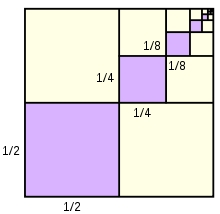Did you find an error or inaccuracy? Feel free to write us. Thank you!

Showing 1 comment:Deas
This app helps so much## Related math problems and questions:

• SequenceIn the arithmetic sequence is a1=-1, d=4. Which member is equal to the number 203?
• 6 termsFind the first six terms of the sequence. a1 = 7, an = an-1 + 6
• Fifth memberDetermine the fifth member of the arithmetic progression, if the sum of the second and fifth members equal to 73, and difference d = 7.
• Arithmetic progression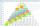In some AP applies: 5a2 + 7a5 = 90 s3 = 12 Find the first member a =? and difference d = ?
• Tenth memberCalculate the tenth member of geometric sequence when given: a1=1/2 and q=2
• SequenceCalculate what member of the sequence specified by (5n-2+15) has value 20.
• Sequence 2Write the first 5 members of an arithmetic sequence a11=-14, d=-1
• SequenceWrite the first 7 members of an arithmetic sequence: a1=-3, d=6.
• SequenceWrite the first 6 members of this sequence: a1 = 5 a2 = 7 an+2 = an+1 +2 an
• Gp - 80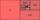One of the first four members of a geometric progression is 80. Find its if we know that the fourth member is nine times greater than the second.
• Difference AP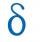Calculate the difference of arithmetic progression if the sum of its first 19 members Sn = 8075 and the first member is a1 = 20
• Geometric sequence 5About members of geometric sequence we know: ? ? Calculate a1 (first member) and q (common ratio or q-coefficient)
• AP - simpleFind the first ten members of the sequence if a11 = 132, d = 3.
• Geometric sequenceIn the geometric sequence is a4 = 20 a9 = -160. Calculate the first member a1 and quotient q.
• GP - sequence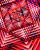The first three terms of a geometric sequence are as follows 10, 30. 90, find the next two terms of this sequence.
• Sequence 11What is the nth term of this sequence 1, 1/2, 1/3, 1/4, 1/5?
• SequenceIn the arithmetic sequence is given: Sn=2304, d=2, an=95 Calculate a1 and n.